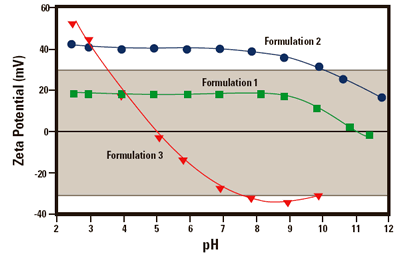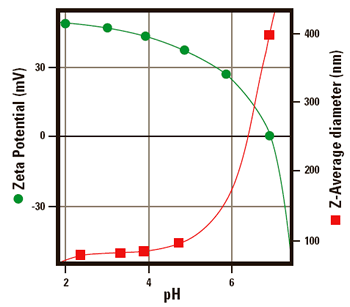# Zeta Potential Measurement Using An Autotitrator And The Effect Of pH

The stability of a particle dispersion will depend upon the balance of the repulsive and attractive forces that exist between particles as they approach one another. If the particles have a mutual repulsion then the particles will remain dispersed.

However, if the particles have little or no repulsive force, then flocculation, aggregation or coalescence will eventually take place.Figure 1. The Malvern Panalytical MPT-1 Autotitrator.

## Zeta Potential Magnitude

The magnitude of the zeta potential of the particles is a measure of this particle interaction.

## Investigating pH and Zeta Potential

One of the most important parameters that determines zeta potential is pH, and the use of an autotitrator such as the Malvern Panalytical MPT-1 is an ideal way of automating the investigation of its effect.

## Effect Of Zeta Potential

The zeta potential of a particle is the overall charge that the particle acquires in a particular medium. The magnitude of the measured zeta potential can be used to predict the long-term stability of the product.

If the particles in a suspension have a large negative or positive zeta potential then they will tend to repel each other and resist the formation of aggregates. However, if the particles have low zeta potential values, i.e. close to zero, then there will be nothing to prevent the particles approaching each other and aggregating.

## Stable and Unstable Particle Suspensions

The dividing line between stable and unstable suspensions is generally taken at either +30 mV or -30 mV. Particles with zeta potentials more positive than +30 mV or more negative than -30 mV are normally considered stable.

## Formulation Changes and Effect On Stability

The effect of changes of the pH, concentration of an additive or the ionic strength of the medium on the zeta potential can give information to aid the formulation of a product to give maximum stability.

## Automating Formulation Changes

The productivity and accuracy of these measurements can be improved by automating the changes to the sample between measurements using an autotitrator. The Malvern Panalytical Multi Purpose Titrator (MPT-1) is capable of performing these titrations in conjunction with a suitable Zetasizer (eg Zetasizer 3000HSA).Figure 2. The zeta potential of 3 formulations of lipid emulsions as a function of pH.

## The Effect Of pH

The most important factor that affects zeta potential is the pH. Many chemically simple surfaces, such as a mineral oxide dispersed in a dilute salt solution, will show a common pattern when the pH is varied. As the pH increases by adding alkali, the surface will become more negative, or at least less positive and the zeta potential will track this.

If acid is added, ionisation will cause the loss of hydroxyl ions which will make the surface more positive. In these simple cases therefore, the pattern is for materials to be more positive at low pH and more negative at high pH. There may be a point where this curve passes through zero zeta potential called the isoelectric point. This is very important for practical purposes as it is normally the pH where the colloidal system is least stable.

## Controlled Flocculation

It should be noted however that contrary to expectations, controlled flocculation is often desirable. If the particles in the dispersion are large and dense enough to sediment, the fact that they repel each other will only ensure sedimentation occurs slowly. The sediment that eventually forms may be dense and difficult to re-disperse. This is the subject of a separate discussion.

## Formulation Example

Figure 2 shows the zeta potential versus pH plots for a series of lipid emulsion samples of different formulation. These measurements were performed on a Zetasizer 3000 HSA in conjunction with the MPT-1. The plots show how the stability of each emulsion formulation is affected by changes in pH.

The shaded area in the diagram (between +30 mV and -30 mV) shows the region in which the sample should be least stable.

## Formulation 1

Formulation 1 is expected to be unstable over the complete pH range. Severe problems with the stability of the sample would be anticipated at the isoelectric point at pH 11.

## Formulation 2

Formulation 2 should remain stable between pH 2 and 10.

However, at pH values higher than 10, flocculation may start to occur.

## Formulation 3

Formulation 3 should be stable at a pH of less than 3.5 or greater than 7.5. Problems in sample stability would be expected to occur between these two pH values.

## Effect On Size

Figure 3 shows the effect of changing the pH on the zeta potential and size of an amidine modified latex. The Z-average diameter is the mean diameter calculated from the Brownian motion of the particles, and is sometimes called the cumulants mean or hydrodynamic diameter.Figure 3. The zeta potential of amidine latex as a function of pH The size of amidine latex as a function of pH.

When the zeta potential is greater than 30 mV (at a pH less than 5) there is sufficient charge present to keep the particles from interacting and the dispersion, and therefore the size of the sample remains stable. However, when the pH is greater than 5, the zeta potential of the sample decreases rapidly and eventually reaches an isoelectric point at pH 7.

The z-average diameter increases as the iso-electric point is approached. This is due to the reduction in repulsive forces between the particles resulting in particle aggregation.

Both of these plots were generated automatically on the same sample using the Zetasizer 3000HSA with the MPT-1 and a sizing flow cell.

The measurements of size and zeta potential were alternated so the size measurement was performed on the same sample that was used to generate the zeta potential data.

## Conclusion

The zeta potential is a parameter that can help predict the stability of a dispersion.

Knowledge of the effect of the pH on the zeta potential of a dispersion can lead to a greater understanding of the mechanisms that affect stability.This information has been sourced, reviewed and adapted from materials provided by Malvern Panalytical.

## Citations

• APA

Malvern Panalytical. (2019, September 03). Zeta Potential Measurement Using An Autotitrator And The Effect Of pH. AZoM. Retrieved on October 21, 2020 from https://www.azom.com/article.aspx?ArticleID=2840.

• MLA

Malvern Panalytical. "Zeta Potential Measurement Using An Autotitrator And The Effect Of pH". AZoM. 21 October 2020. <https://www.azom.com/article.aspx?ArticleID=2840>.

• Chicago

Malvern Panalytical. "Zeta Potential Measurement Using An Autotitrator And The Effect Of pH". AZoM. https://www.azom.com/article.aspx?ArticleID=2840. (accessed October 21, 2020).

• Harvard

Malvern Panalytical. 2019. Zeta Potential Measurement Using An Autotitrator And The Effect Of pH. AZoM, viewed 21 October 2020, https://www.azom.com/article.aspx?ArticleID=2840.